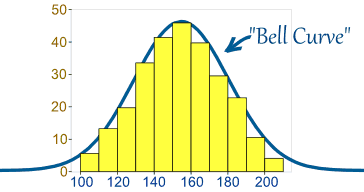## Friday, September 09, 2016

### What Statistical Tests do Data Analysts need to know? #114

So you want to use statistics to help make sense of data? Where do you start?

What are the following tests used for?:

• Student t-Test
• Mann-Whitney U-Test
• Shapiro-Wilk Test
• Multiple Regression
• ...and many moreImage source: Math is Fun.
Each statistical test has a specific purpose. But most rely on some assumptions - the critical one being are the data normal. By this I mean that if you plot all the values in a histogram, would the chart look like a "bell" curve - this is what you expect most of the time (ie normal). Take a group of 100 males and measure all their heights. If the sample of 100 males was taken randomly from a population you should end up with a few short guys, a few tall guys, but most would be in-between. You would end up with a chart that should look like the one on the right. If this is the case you can assume that the data are normal. If the distribution (the bit in yellow) is skewed to the left or right - you cannot assume that the data are normal.

So - if for example you wanted to compare two samples to see if there is a significant difference between the two groups, you first have to decide if both samples are normal (ie - fit a bell curve). You could also see if the mean, median, and mode are close in value - this is also a very good rule of thumb to tell us if the data are normal. If the data are normal, you use Student's t-Test to see if there is a significant difference between the two group, if the data are not normal, you should use the Mann-Whitney U Test. Most of the time we will be using the t-Test, especially if we have large sample (greater than 30 values) - but it is important to choose the correct test in the first place.

Sounds complicated?

Many College students have a statistics module somewhere along their journey to a degree, and they will learn how to perform these tests. Sometimes they will learn how to do the tests by hand, or use a tool such as SPSS.

Now let's put some context on this. Suppose you wanted to compare the results of two drug trials where two separate drugs are being tested to cure a disease. The results of such a test could be vital to the health of future generations, and also to the bank balance of the drug company. So they will want to know for sure if there is a significant difference or not between the drugs. Hence the need to use a statistical test (in this case a Student t-Test) to make the inference. Nobody wants a drug injected into their body that has not been tested fully. How accurate is the test? Can we say with at least 99% certainty that the result is a correct one? Only statistics will tell you this.